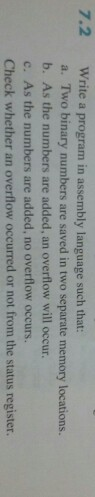# Question & Answer: Write a program in assembly language such that: a. Two binary numbers are saved in two separate memory…..Write a program in assembly language such that: a. Two binary numbers are saved in two separate memory locations. b. As the numbers are added, an overflow will occur. c. As the numbers are added, no overflow occurs. Check whether an overflow occurred or not from the status register.

(a)

MVI A, 2H ;Copy value 2H in register A

Don't use plagiarized sources. Get Your Custom Essay on
Question & Answer: Write a program in assembly language such that: a. Two binary numbers are saved in two separate memory…..
GET AN ESSAY WRITTEN FOR YOU FROM AS LOW AS \$13/PAGE

MVI B, 4H ;Copy value 4H in register B

```DATA SEGMENT
NUM1 DB 8H
NUM2 DB 8H
SUM DB ?
ENDS

CODE SEGMENT
ASSUME DS:DATA CS:CODE
START:
MOV AX,DATA
MOV DS,AX

MOV AL,NUM1

MOV SUM,AL

INT 21H
ENDS
END START
```

(b)

```If the sum of two numbers with the sign bits off yields a result number
with the sign bit on, the "overflow" flag is turned on.

0100 + 0100 = 1000 (overflow flag is turned on)

If the sum of two numbers with the sign bits on yields a result number
with the sign bit off, the "overflow" flag is turned on.

1000 + 1000 = 0000 (overflow flag is turned on)
```

(c)

```Otherwise, the overflow flag is turned off.
* 0100 + 0001 = 0101 (overflow flag is turned off)
* 0110 + 1001 = 1111 (overflow flag is turned off)
* 1000 + 0001 = 1001 (overflow flag is turned off)
* 1100 + 1100 = 1000 (overflow flag is turned off)

We only need to look at the sign bits (leftmost) of the three
numbers to decide if the overflow flag is turned on or off```